Courses
Courses for Kids
Free study material
Free LIVE classes
More

# Properties of AltitudeLIVE
Join Vedantu’s FREE Mastercalss

## Overview of Properties of Altitude

Have you ever wondered what that line which falls perpendicular to either of the sides of a triangle is called? Or ever wondered if there can only be one of those lines in a figure or if there can be even more? These perpendicular lines coming from the vertex of any triangle and then falling perpendicularly on the opposite side are called altitude in mathematics. In this article, we will look at these altitudes, will also learn the geometrical property of altitudes, and at last will also learn what is an orthocentre of a triangle. Sounds interesting right? So let's start learning.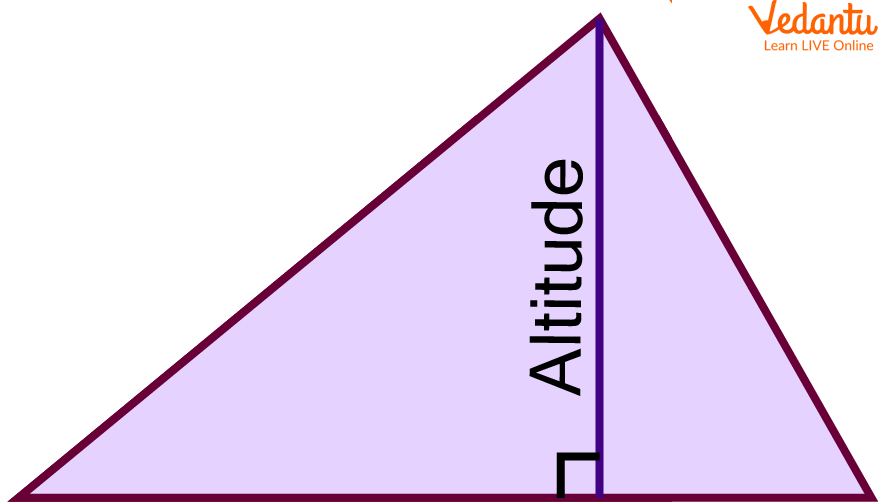Altitude of a Triangle

### What Is Altitude?

In a triangle, a particular Line segment which is drawn from its vertex and is connected to the opposite side of the triangle making a 90-degree inclination with that side is referred to as the altitude.

Triangles usually are of three kinds of obtuse, equilateral, and isosceles triangles.

In each type of triangle, the property of their altitude differs in various ways and usually is used to calculate the area of a triangle because the altitude is equivalent to the height of the triangle.

• In an obtuse-angled triangle the altitude is present outside the main triangle body for which we have to extend the base of the triangle and then draw a perpendicular line segment from the opposite vertex touching the extended base

• The altitude in an equilateral triangle interestingly divides the triangle into two equal parts.

• The isosceles triangle altitude bisects the angle of the vertex and bisects the base. It should be noted that an isosceles triangle is a triangle with two congruent sides and so, the altitude bisects the base and vertex.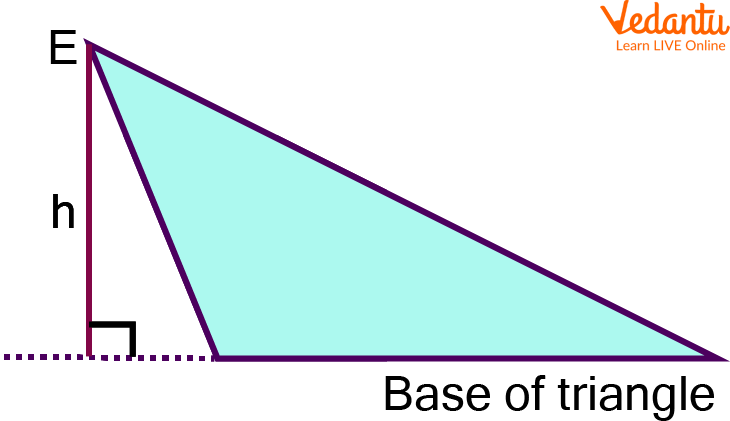Altitude of an Obtuse-Angled Triangle

## Properties of Altitude

Below are listed some properties of altitude:

• There can only be a maximum of three altitudes inside or outside a triangle.

• It is at a 90 degrees angle to the opposite side.

• The altitude of a triangle can either be inside it or can also be outside it depending upon the type of triangle being talked about.

• As we all know, there can only be a max of three altitudes inside a triangle. Therefore, the junction at which all those three altitudes meet is referred to as the orthocentre of a triangle.

## Properties of Orthocentre

In this section of the article, we will discuss about the various properties of orthocenter:

As discussed in the above section a triangle can only have a maximum of three altitudes and the portion at which those three altitudes meet with one another is called an Orthocentre. Now we will look at the orthocenter of a triangle properties: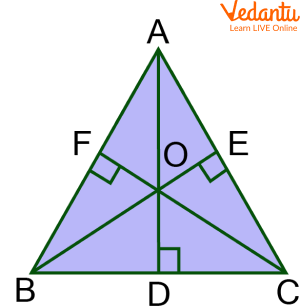The point indicated as ‘O’ is the orthocentre of a right-angled triangle

• For an acute angled Triangle, the orthocentre is drawn inside the triangle body.

• For an obtuse-angled triangle, the orthocentre is drawn outside the triangle body

• Whereas the orthocentre of a right-angled triangle is drawn at the vertices of the right angles.

Above were some unique orthocenters of a triangle properties.

## Formulas to Calculate the Altitudes of Various Triangles

 Type of triangle Formula for calculating altitude Isosceles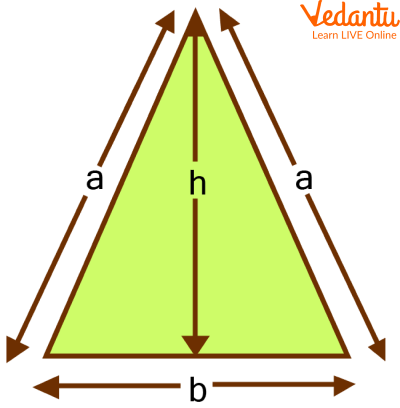$h=\sqrt{a^2-\dfrac{b^2}{4}}$ where ‘a’ is the length of the two equal sides and ‘b’ is the other side. Equilateral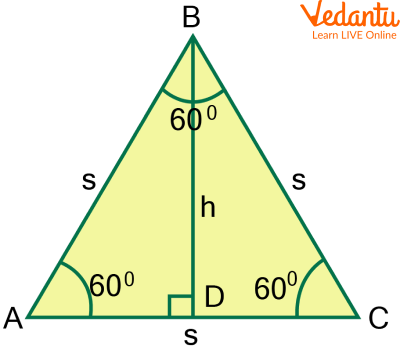$h=\dfrac{s \sqrt{3}}{2}$ where ‘s’ is the length of the side Right angle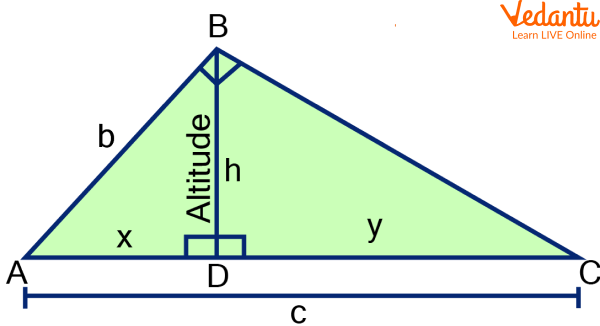$h=\sqrt{x y}$ where ‘x’ and ‘y’ are the measures of the base as divided by the altitude falling on the base.

## Geometrical Property of Altitudes

Below are listed the geometrical properties of altitudes

1. All of the altitudes in a triangle are concurrent or congruent.

2. The altitude of a triangle lies inside or outside the triangle.

3. Orthocentre can either lie inside or outside the triangle

## Solved Examples

Below are some questions related to the altitude of a triangle:

Example 1: Calculate the altitude of a triangle having all equal sides of measure 6 cm.

Ans: According to the question the given triangle is an equilateral triangle

The formula for calculating the altitude of an equilateral triangle is $h=\dfrac{1}{2} \times \sqrt{3} \times s$ where $s$ is the length of the sides.

Therefore, $h=\dfrac{1}{2} \times \sqrt{3} \times 6$

$h=3 \sqrt{3} \mathrm{~cm}$

Example 2: Calculate the side of the equilateral triangle if the height or the altitude is 2cm.

Ans: For equilateral triangle

$h$ (height or altitude) $=\dfrac{1}{2} \times \sqrt{3} \times s$ where ' $s$ ' is the length of the side Given $h=2 \mathrm{~cm}$ noe putting the value in the equation we get

$(h \times 2) \div \sqrt{3}=s$

$(2 \times 2) \div \sqrt{3}=s$

Therefore, $\mathrm{s}=\dfrac{4}{\sqrt{3}} \mathrm{~cm}$

## Practice Questions

Q 1. Write down all the formulas for finding altitude of the following triangles:

1. Right angle triangle

2. Isosceles triangle

Ans: a. $h=\sqrt{x y}$

b. $h=\sqrt{a^2-\dfrac{b^2}{4}}$

Q 2. Find the measure of the altitude of a triangle having three equals sides of 8 cm

Ans: $4 \sqrt{3} \mathrm{~cm}$

Q 3. Calculate the height of a right angle triangle whose base values are $x=2 \mathrm{~cm}$ and $y=4 \mathrm{~cm}$

Ans: For right angle triangle $h=\sqrt{xy}$

Therefore, $h=\sqrt{2 \times 4}=2 \sqrt{2} \mathrm{~cm}$

## Summary

To complete all the learnings from this article we can say that altitudes are the heights of a triangle and are usually used to calculate the area of the triangle. Altitudes can help us find and relate various triangle properties with each other for example congruency. Through this article, we learned about the basic properties of altitudes of the triangle and also looked at the positions of the altitudes. With this, we would like to end this article and hope that we were clear and understandable enough to clear all your doubts yet if you still have doubts you can write them down in the comment section below.

Last updated date: 27th Sep 2023
Total views: 78.3k
Views today: 2.78k

## FAQs on Properties of Altitude

1. Are there any differences between the orthocentre and centroid of a triangle

The only main difference is that the orthocentre is the point where all the altitudes meet whereas the centroid is the point from which the line segments drawn from the center of each side of a triangle meet.

2. Are altitude and median the same?

No they are different median join the vertex to the center whereas altitude passes through vertex and join to opposite side.

3. Name all types of triangles.

Equilateral, isosceles, scalene, right angles, acute, obtuse are various types of triangle.